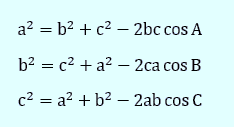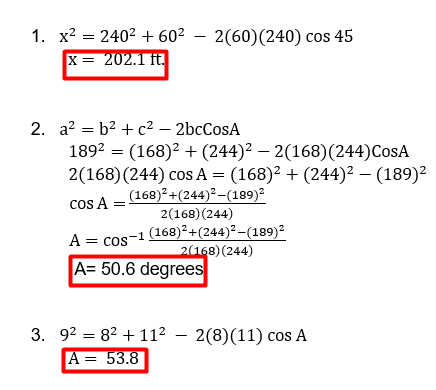# Law of Cosines Word Problems Worksheet (with answer key)

The cosine of one of a triangle’s angles and the lengths of its sides are related by the law of cosines. We are now able to calculate angles and distances that are impossible to measure without the aid of trigonometry. The law of cosines is used to compute the third side of a triangle when we know the first two sides and the enclosed angle, as well as to compute the angles of a triangle when we know all three sides.

What is the “Law of Cosines Word Problems Worksheet (with answers)”?

This worksheet will explore some of the Law of Cosines Word Problems Worksheet.

## What is the Law of Cosines?

A triangle’s side lengths and the cosine of its angles can be related to one another using the law of cosine. The Pythagoras theorem, which is applicable to a right triangle, is generalized by the cosine law in trigonometry.

This worksheet will help you to examine the law of the cosines formula and its origin before using the cosine function to investigate how these elements interact.

Instructions on how to use the “Law of Cosines Word Problems Worksheet (with answers).”

Examine the concept and examples provided, then attempt to complete the exercises below.

## Conclusion

According to the law of cosine, the square of any one side of a triangle equals the difference between the sum of the squares of the other two sides and double the product of the other sides and the cosine angle added between them.

If you have any inquiries or feedback, please let us know.

## Law of Cosines Word Problems Worksheet (with answer key)

Law of Cosines Definition

The square of any one side of a triangle is equal to the difference between the sum of the squares of the other two sides and double the product of the other sides and the cosine angle included between them, according to the law of cosine. The cosine rule is another name for the cosine law. Any triangle can benefit from using this law to fill in the blanks.

Law of Cosines Formula

When a triangle’s two sides and included angles are known, the law of cosines formula can be used to determine the missing side.Finding Missing Length and Angles Using the Law of Cosines

To comprehend how to use the cosine law to find a triangle’s missing side or angle, let’s look at the subsequent steps.

• Step 1: Write down the triangle’s side lengths and angle measurements as well as the element that needs to be calculated.
• Step 2: Apply the cosine rule formulas,
a2 = b2 + c– 2bc·cosA
b2 = c2 + a2 – 2ca·cosB
c2 = a2 + b2 – 2ab·cosC
where, A, B, and C are the vertices of a triangle, and their opposite sides are represented as a, b, and c respectively.
• Step 3: Express the result that was reached in the appropriate units.

For example.

Two sides of a triangle measure 72 in and 50 in with the angle between them measuring 49º let us find the missing side.

The pitcher’s mound on a women’s softball is 43 feet from home plate and the distance between the bases is 60 feet, as shown in figure 6.8. The pitcher’s mound is not halfway between home plate and second base. How far is the pitcher’s mound from the first base?

Solution:

1. Law of Cosines Word Problems
1. In a softball, a batter hits a ball to the dead center field, a distance of 240 feet from home plate. The center fielder then throws the ball to third base and gets a runner out. The distance between the bases is 60 feet. How far is the center fielder from third base?1. Two airplanes leave an airport at the same time. An hour later they are 189 km apart. If one plane traveled 168 km and the other plane traveled 244 km during the hour, find the angle 0 between their flight paths.1. Joe is on vacation at a tropical bay that has three islands. He rents a boat on Island A and plans to navigate to Island C, which is 8 miles away. Based on the figure below, at what angle 0 should he navigate to go to the island?